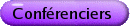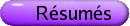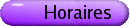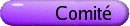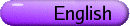Positivité an analyse fonctionnelle et applications
Org: Charalambos Aliprantis (Purdue) et Vladimir Troitsky (Alberta)
[PDF]

SAFAK ALPAY, Middle East Technical University, Turkiye
On b-weakly compact operators
[PDF]

A subset A of a vector lattice E is called b-bounded if A is bounded in E ~ ~ , the order bidual of E. An operator T: E ® X, where E is a Banach lattice and X is a Banach space, is called b-weakly compact if for each b-bounded subset A in E, T(A) is relatively weakly compact in X. We will give some recent results on b-weakly compact operators. In particular, we will give a dual characterization of b-weakly compact operators and investigate factorization properties of such operators.

Unconditional decompositions in Banach spaces
[PDF]

We discuss properties related to unconditionality in Banach spaces which admit a UFDD (unconditional finite-dimensional decomposition). As a consequence we obtain that if a Banach space X contains an unconditional basic sequence then we have one of the following "regular-irregular" alternatives: either X contains a subspace isomorphic to l2 or X contains a subspace which has a UFDD but does not admit a UFDD with a uniform bound for the dimensions of the decomposition. This result can be also viewed in the context of Gowers' dichotomy theorem.

EVGENIOS AVGERINOS, University of the Aegean, Rhodes, Greece
On the solutions of multivalued problems governed by vector differential inclusions
[PDF]

In this work we consider second order vector differential inclusions with periodic boundary conditions and a general multivalued term. So let T=[0,b]. The multivalued boundary value problem under consideration is the following:

ì
ï
í
ï
î
 x¢¢(t) Î F æè t,x(t),x¢(t) öø a.e. on T
 x(0)=x(b),  x¢(0) = x¢(b)
ü
ï
ý
ï
þ
\tag1
(1)

Second order differential inclusions have been studied recently primarily with Dirichlet boundary conditions and with a multivalued term which is compact, convex valued and satisfies a growth condition. There have been some works where the convexity hypothesis on the values of the multifunction has been dropped and/or the Dirichlet boundary condition has been replaced by a more general nonlinear one. This development of the research on second order multivalued boundary value problems, can be traced in recent works. In the paper we combine the method of Hu-Papageorgiou with techniques from the theory of nonlinear operators and the multivalued Leray-Schauder principle, to establish the existence of a solution for problem (1) under very general hypotheses on F(t,x,y).

GERARD BUSKES, University of Mississippi
Geometric mean in vector lattices
[PDF]

We compare the geometric mean and the square mean in vector lattices and show that the geometric mean enables one to define the square of an Archimedean vector lattice.

ROMAN DRNOVSEK, Institute of Mathematics, Physics and Mechanics; University of Ljubljana, Jadranska 19, SI-1000 Ljubljana, Slovenia
On positive unipotent operators on Banach lattices
[PDF]

An operator on a Banach space is said to be unipotent whenever its spectrum contains only the number 1. Let T be a positive unipotent operator on a complex Banach lattice. Huijsmans and de Pagter posed a question whether T is necessarily greater than or equal to the identity operator I. We give a partial answer to the question by proving that this is true if limn®¥ n||(T-I)n||1/n = 0.

EDUARD EMELYANOV, Middle East Technical University, Ankara, Turkey
Some recent results on constrictive operators
[PDF]

Let X be a Banach space. An operator T Î L(X) is called constrictive if there exists a compact A Í X such that

 lim n®¥ dist(Tn x,A) = 0     ("x Î X, ||x|| £ 1).
T is called quasi-constrictive if its stable space
 X0 (T) = {x Î X : lim n®¥ ||Tn x|| = 0}
is closed and of finite co-dimension. We discuss some conditions on T under which T is constrictive or quasi-constrictive.

Domination by positive Banach-Saks operators
[PDF]

Given a positive Banach-Saks operator T between two Banach lattices E and F, we give sufficient conditions on E and F in order to ensure that every positive operator dominated by T is Banach-Saks. A counterexample is also given when these conditions are dropped. Moreover, we deduce a characterization of the Banach-Saks property in Banach lattices in terms of disjointness.

VALENTINA GALVANI, University of Alberta, Department of Economics
Spanning with Options over a Borel Space
[PDF]

The research question is whether portfolios of finitely many ordinary call options (or put options) allow to hedge any financial claim. The situation considered is a two-date incomplete securities market defined over a metrizable state-space W containing uncountably many states of nature. The investigation is limited to securities markets for which the space of contingent claims is identified with an Lp-space, with 1 £ p < ¥, on the Borel space (W,B,P) where B is the Borel s-algebra of W and P is a nonatomic Borel regular probability.

A claim is an element of the space of contingent claims. The constant function 1 represents the riskfree asset payoff. The expression (s-k1)+ describes the payoff of a call option written on an underlying asset s with strike price k. The payoff of a portfolio of the riskfree asset and of finitely many call options is an element of the space Os defined by

 Os = Span{1, (s-k1)+ : k Î Â}.
Options are said to span the market if the space Os is dense in the space of contingent claims. This paper proves that there exist infinitely many underlying assets for which options span a separable Lp-space. In particular, if W is also Polish, this collection of underlying assets is dense in the positive cone of Lp(P).

HAILEGEBRIEL GESSESSE, University of Alberta, Edmonton, AB
Minimal Vectors of Positive Operators On Ordered Banach Spaces
[PDF]

We extend the technique of minimal vectors to lattice-ordered Banach Spaces which are not Banach Lattices, but with norm that satisfy | |x| | = |x| for every x. We prove the existence of invariant subspaces for some positive operators on these spaces. As a special case, we consider operators on Sobolev Spaces.

NIGEL KALTON, University of Missouri
Symmetric functionals and traces
[PDF]

We will discuss some problems concerning the existence of symmetric or rearrangement-invariant linear functionals on sequence and function spaces, with applications to non-standard traces on ideals of operators.

This work includes joint work with Fyodor Sukochev and with Ken Dykema.

Universally order bounded operators on Hilbert Spaces
[PDF]

It is shown that linear bounded operators on a Hilbert space H which are regular for any representation of H as a L2 are exactly the sums of Hilbert-Schmidt operators and multiples of the identity operator.

MEHMET ORHON, University of New Hampshire, Mathematics Department, Kingsbury Hall, Durham, NH 03824
On the ideal center of the dual of a Banach lattice
[PDF]

Let X be a Banach lattice. Its ideal center Z(X) is embedded naturally in the ideal center Z(X¢) of its dual. The embedding may be extended to a contractive algebra and lattice homomorphism of Z(X)" into Z(X¢). We show that the extension is onto Z(X¢) if and only if X has a topologically full center (that is, the closure of Z(X)x is the closed ideal generated by each x Î X). The result can be generalized to the ideal center of the order dual of an Archemedian Riesz space and in a modified form to the orthomorphisms on the order dual of an Archemedian Riesz space.

HEYDAR RADJAVI, University of Waterloo, Waterloo, Ontario N2L 3G1
Invariant Sublattices for Semigroups of Operators
[PDF]

Let X be a Banach lattice and S a set of operators on X. This is a report on joint work in progress with Vladimir Troitsky concerning those sublattices of X which are invariant under every member of S. We study conditions under which nontrivial invariant sublattices exist.

ANTON SCHEP, University of South Carolina, Columbia, SC 29208, USA
Products of (weak) log convex operators are log convex
[PDF]

A family {A(t);t Î I} of positive operators on a Banach lattice E is called (weak) log convex if the function x® áA(t)x, x* ñ is log convex for all 0 £ x Î E and all 0 £ x* Î E*. The main result is that the operator product of two such families is again (weak)log convex. Applications to Kingman's Theorem about log convexity of the spectral radius of such families follow.

On the existence of asymptotic-lp structures in Banach spaces
[PDF]

The asymptotic theory of infinite dimensional Banach spaces, developed by Maurey, Milman and Tomczak-Jaegermann, is concerned with the structure of infinite dimensional Banach spaces manifested in the finite-dimensional subspaces that appear everywhere far away in the space. The class of spaces that have a simple asymptotic structure, in the sense that we can find a 1 £ p £ ¥ such that all such finite-dimensional subspaces as before are essentially lpn's, are of special interest and they are called asymptotic-lp spaces.

We prove that if a Banach space is saturated with infinite dimensional subspaces in which all special n-tuples of vectors are equivalent, uniformly in n, then the space contains asymptotic-lp subspaces, for some 1 £ p £ ¥. The proof reflects a technique used by Maurey in the context of unconditional basic sequence problem and extends a result by Figiel, Frankiewicz, Komorowski and Ryll-Nardzewski.

VLADIMIR TROITSKY, University of Alberta, Edmonton, Alberta T6G 2G1
Norm closed algebraic ideals in L(lp Ålq)
[PDF]

It is well known that the only proper non-trivial norm-closed algebraic ideal in the algebra L(X) for X = lp (1 £ p < ¥) or X=c0 is the ideal of compact operators. The next natural question is to describe all closed ideals of L(lp Ålq) for 1 £ p,q < ¥, p ¹ q, or, equivalently, the closed ideals in L (lp,lq) for p < q. We show that for 1 < p < 2 < q < ¥ there are at least four distinct proper closed ideals in L (lp,lq), including one that has not been studied before.

This is a joint work with B. Sari, Th. Schlumprecht and N. Tomczak-Jaegermann.

MARTIN WEBER, Technische Universität Dresden
On Finite Elements in Vector Lattices of Operators
[PDF]

In Archimedean vector lattices the notion of a finite element simulates a continuous function with compact support in abstract setting. Besides exhaustive studies of finite elements, in particular vector lattices and Banach lattices, the investigation of finite elements in vector lattices consisting of operators is also of interest and yields some interesting results. The main results up to now are obtained for the vector lattice of all regular operators between two vector lattices. So the orthomorphisms in and the lattice isomorphisms between Dedekind complete Banach lattices are finite elements. If E,F are Banach lattices with F reflexive, then an operator is a finite element if and only if its adjoint is finite. Any regular operator defined on an AL-space which has its images in a Dedekind complete AM-space with order unit is a finite element. Vice versa, if for two Banach lattices E,F with F Dedekind complete each regular operator mapping E into F is a finite element, then E is lattice isomorphic to an AL-space and F lattice isomorphic to an AM-space (not necessary with order unit). Not each finite rank operator is a finite element. However, if the constitutes of such an operator are finite elements in their corresponding vector lattices then the operator is a finite element.